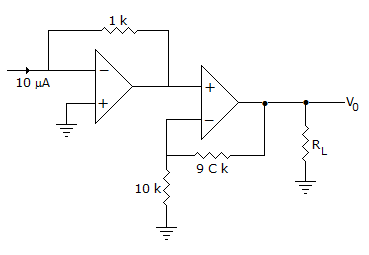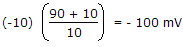# Electronics and Communication Engineering - Analog Electronics - Discussion

16.

The output V0 in figure is[A]. -100 V [B]. -100 mV [C]. 10 V [D]. 10 mV

Explanation:

Input to non-inverting op-amp is -10 x 10-6 x 103 = -10 mV.

Therefore output == -100 mV.

 Mianl said: (Oct 4, 2014) What about first inverting amplifier?

 Manjappa Hosakeri said: (Jan 9, 2015) First inverting amplifier is op-amp is -10 x 10-6 x 103 = -10 mV.

 Mukesh said: (Feb 8, 2015) Please tell 2nd step how -100mv will come?

 Bindu said: (Mar 18, 2015) What is the formulae used for to get output voltage?

 Vim said: (May 26, 2015) Anyone can please explain the solution?

 Roopak said: (Jun 19, 2015) vo = vin(1+R1/R2) where vin = -10mv, R1 = 90, R2 = 10.

 Ritesh Maurya said: (Jun 20, 2015) Second stage is nothing it just out put of non inverting opamp. Vout = (1+rf/r1)*Vinput. Vout = (1+90/10)*-10.

 Jyothi R said: (Oct 4, 2016) Let first op amp output is v01; By using KCL ((0 - v01)/(1k)) = (10 micro amps); v01 = -10mV; v01 is the input to the second op amp that to non inverting. If we give non-inverting input, here output will be (1 + (R2/R1)v01; So, v0 = (1 + (90/10))(-10mV) = -100mV.

 Mukund said: (Jun 1, 2017) I think the answer is option A.

 Vishakadatta said: (Jul 23, 2017) @Mukund. There is milli(m), so its 100mV.